# Chapter 4: Geometry - Online Test

Q1. If in triangles ABC and EDF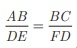then they will be similar, when
Explaination / Solution:Q2. In ΔLMN, L = 60°,  M = 50° . If ΔLMN ~ ΔPQR then the value of R is
Explaination / Solution:Q3. If ΔABC is an isosceles triangle with ∠C = 90° and AC = 5 cm, then AB is
Explaination / Solution: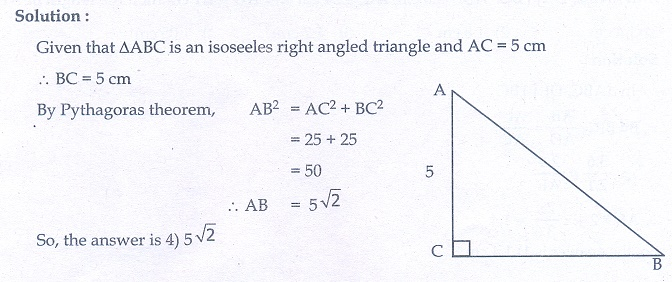Q4.

In a given figure ST || QR, PS = 2 cm and SQ = 3 cm. Then the ratio of the area of ΔPQR to the area of ΔPST is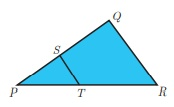Explaination / Solution: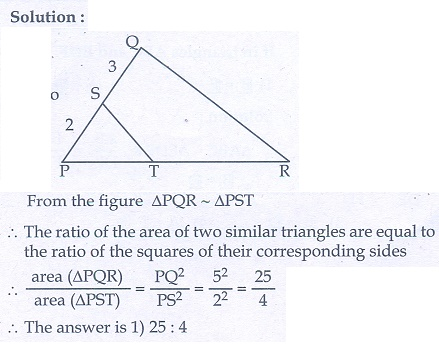Q5. The perimeters of two similar triangles ΔABC and ΔPQR are 36 cm and 24 cm respectively. If PQ = 10 cm, then the length of AB is
Explaination / Solution: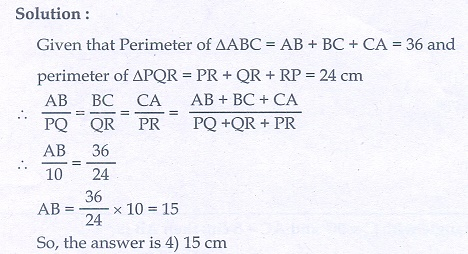Q6. If in ΔABC , DE || BC . AB = 3.6 cm, AC = 2.4 cm and AD = 2.1 cm then the length of AE is
Explaination / Solution:Q7. In a ΔABC , AD is the bisector of ∠BAC . If AB = 8 cm, BD = 6 cm and DC = 3 cm. The length of the side AC is
Explaination / Solution:Q8.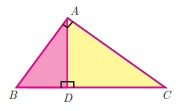Explaination / Solution:Q9. Two poles of heights 6 m and 11 m stand vertically on a plane ground. If the distance between their feet is 12 m, what is the distance between their tops?
Explaination / Solution: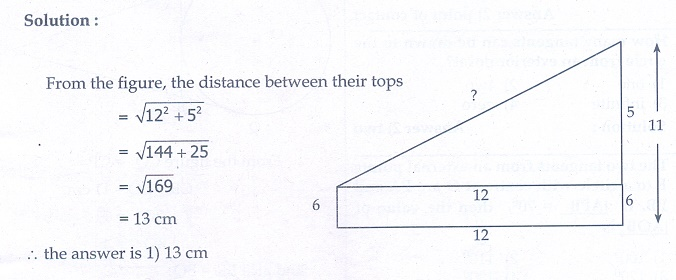Q10.

In the given  figure,  PR = 26 cm,  QR = 24  cm. PAQ = 90° , PA=6 cm and QA = 8 cm. Find PQRExplaination / Solution: